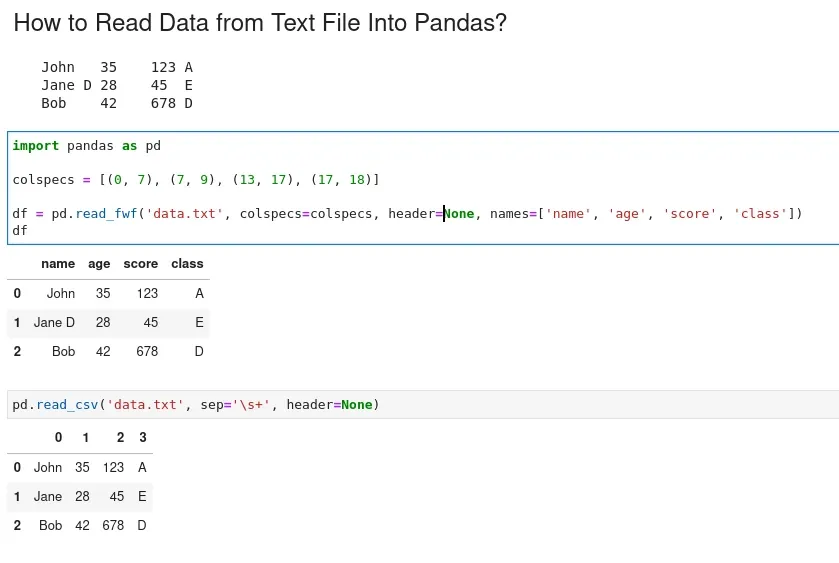The following step-by-step example shows how to load data from a text file into Pandas. We can use:

• `read_csv()` function
• it handles various delimiters, including commas, tabs, and spaces
• `pd.read_fwf()`
• read fixed-width formatted lines into DataFrame

Let's cover both cases into examples:

To read a text into Pandas DataFrame we can use method `read_csv()` and provide the separator:

``````import pandas as pd
``````

Where `sep` argument specifies the separator. Separator can be continuous - `'\s+'`.

Other useful parameters are:

• `header=None` - does the file contain headers
• `names=["a", "b", "c"]` - the column names
• `skiprows=[0,1]` - skip rows
• `index_col=True` - use index from the file

To read data from a fixed-width file in Pandas we can use read_fwf. Suppose we have a file `'data.txt'` like:

``````John   35	123 A
Jane D 28	45  E
Bob	42	678 D
``````

We can see that columns are aligned by position rather than separated by delimiters. Since the columns are separated by fixed widths:

• first column - 7 chars
• (0, 7)
• second - 2 chars
• (7, 9)

we can't use `read_csv()` with a separator. Instead we will:

• specify the column widths
• read the fixed-width file into a DataFrame
``````import pandas as pd

colspecs = [(0, 7), (7, 9), (13, 17), (17, 18)]

df
``````

The result is:

name age score class
0 John 35 123 A
1 Jane D 28 45 E
2 Bob 42 678 D## Pandas read text file line by line

To read a text file line by line into a pandas DataFrame we can:

• create an empty DataFrame
• create an iterator to read the file line by line
• iterate over the iterator and append each line to the DataFrame
• reset the index of the DataFrame
``````import pandas as pd

df = pd.DataFrame()

for chunk in iterator:
df = df.append(chunk)

df = df.reset_index(drop=True)
``````

## Pandas read text file with pattern

As an alternative we can use list comprehension to read files and filter it.

Let's work with the following file:

``````John   35	123 A
Pattern
Jane D 28	45  E
Bob	42	678 D
End of pattern
``````

We can find the numbers of the start and end lines by matching pattern:

``````a=[]
with open('data.txt',"r") as r:
a=[x.replace("\n","") for x in a]
start = a.index("Pattern") +1
end = a.index("End of pattern")
start, end
``````

After that we can read the file with `read_fwf` or `read_csv` and filter the lines:

``````import pandas as pd

df = df[start:end]
``````

Which give us:

name age score class
2 Jane D 28 45 E
3 Bob 42 678 D

## Summary

We've seen three different ways of reading and loading text file into Pandas DataFrame. We covered how to read delimited or fixed-length files with Pandas.

We also saw how to read text files line by line and how to filter csv or text file by pattern.